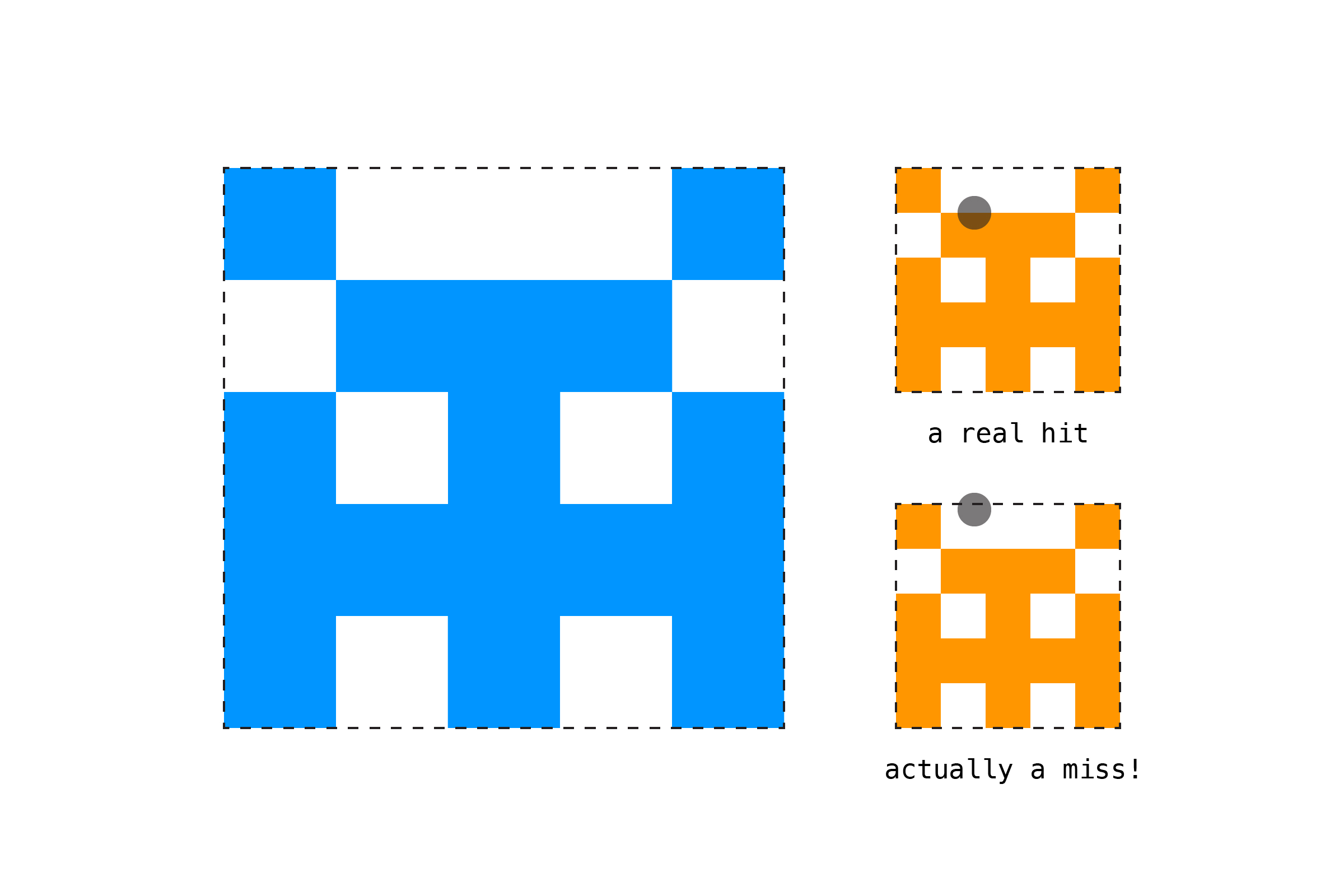[ your browser does not support the canvas tag ]

# RECTANGLE/RECTANGLE

Moving from Point/Rectangle to two rectangles is easy, but the if statements start to get pretty long. Let's say we have two squares, `r1` and `r2`, with positions and sizes set like the last example. We now have to check:

``````Is the RIGHT edge of r1 to the RIGHT of the LEFT edge of r2?
Is the LEFT edge of r1 to the LEFT of the RIGHT edge of r2?
Is the BOTTOM edge of r1 BELOW the TOP edge of r2?
Is the TOP edge of r1 ABOVE the BOTTOM edge of r2?
``````

Yeah, not so intuitive 😖. A picture will probably help:To start, let's test the right edge of `r1` with the left edge of `r2`:

``````float r1RightEdge = r1x + r1w;
if (r1RightEdge >= r2x) {
// right edge of r1 is past left edge of r2
}
``````

We can expand this idea, checking all four edges:

``````if (r1x + r1w >= r2x &&     // r1 right edge past r2 left
r1x <= r2x + r2w &&       // r1 left edge past r2 right
r1y + r1h >= r2y &&       // r1 top edge past r2 bottom
r1y <= r2y + r2h) {       // r1 bottom edge past r2 top
return true;
}
return false;
``````

While the math here is simple addition, this is the trickiest collision for most people to get used to. With practice, you'll be able to picture this in your head. Of course, building a re-usable function makes checking for collisions much easier! In the meantime, it may help to map things out on a piece of paper when you're writing your code.

Here's a full example:

``````float s1x = 0;      // square position (move with mouse)
float s1y = 0;
float s1w = 30;     // and dimensions
float s1h = 30;

float s2x = 200;    // same for second square
float s2y = 100;
float s2w = 200;
float s2h = 200;

void setup() {
size(600,400);
noStroke();
}

void draw() {
background(255);

// update square to mouse coordinates
s1x = mouseX;
s1y = mouseY;

// check for collision
// if hit, change rectangle color
boolean hit = rectRect(s1x,s1y,s1w,s1h, s2x,s2y,s2w,s2h);
if (hit) {
fill(255,150,0);
}
else {
fill(0,150,255);
}
rect(s2x,s2y, s2w,s2h);

// draw the other square
fill(0, 150);
rect(s1x,s1y, s1w,s1h);
}

// RECTANGLE/RECTANGLE
boolean rectRect(float r1x, float r1y, float r1w, float r1h, float r2x, float r2y, float r2w, float r2h) {

// are the sides of one rectangle touching the other?

if (r1x + r1w >= r2x &&    // r1 right edge past r2 left
r1x <= r2x + r2w &&    // r1 left edge past r2 right
r1y + r1h >= r2y &&    // r1 top edge past r2 bottom
r1y <= r2y + r2h) {    // r1 bottom edge past r2 top
return true;
}
return false;
}
``````

It's worth noting two important things here. First, the last two examples use squares, but any rectangle will work with this code. Second, this algorithm assumes you're using the default `rectMode(CORNER)`, which draws rectangles from the top corner and specifies width/height. If you want to use `rectMode(CENTER)`, you'll need to modify this algorithm (see the Challenge Questions at the end of this section).Similar to the Circle/Circle example, Rectangle/Rectangle collision can be used to draw "bounding boxes" around more complex shapes. However, what you gain in performance you lose in accuracy. If you've ever played a game and frustratedly shouted "I totally hit you!" you have probably experienced bounding boxes that don't quite line up with their objects. Find the right balance between actually being correct and what feels right for the user.

NEXT: Circle/Rectangle